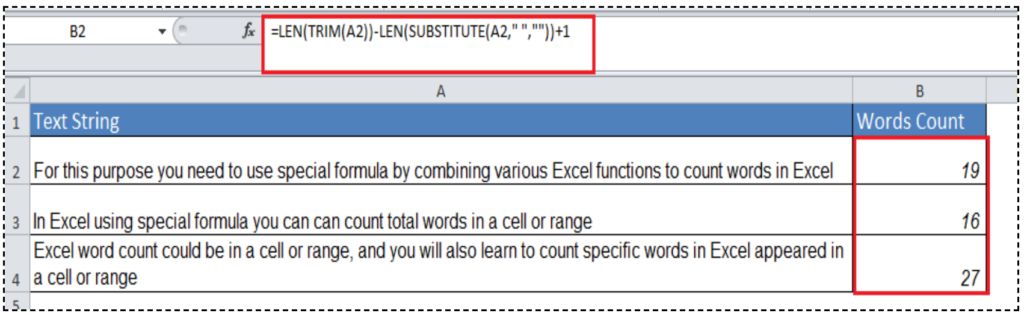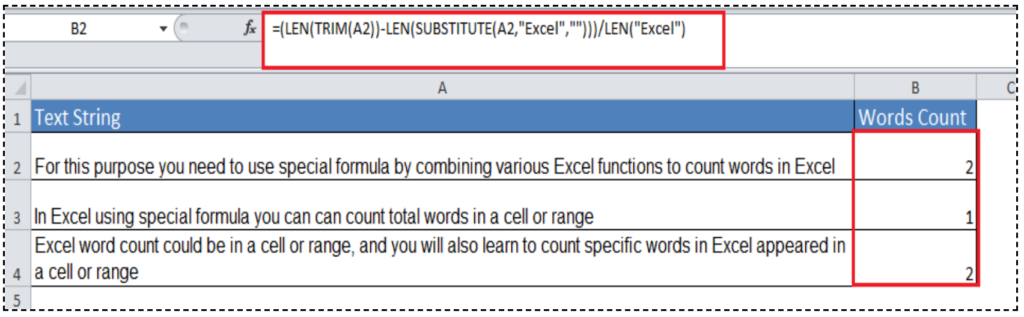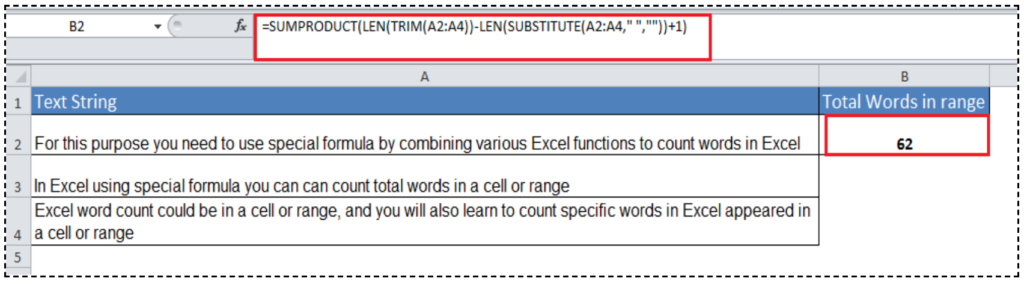Get instant live expert help with Excel or Google Sheets“My Excelchat expert helped me in less than 20 minutes, saving me what would have been 5 hours of work!”

#### Post your problem and you’ll get expert help in seconds.

Your message must be at least 40 characters
Our professional experts are available now. Your privacy is guaranteed.

# How to Count Words in Excel

Excel has built-in functions to count cells based on various condition(s). These include cells containing specific values like numbers, text, blank or non-blank values, and to calculate the length of a string. But unfortunately, there is no special built-in function to count words in Excel. This is possible, however, with the right formula.

## How to count words in Excel

To count words in Excel, you need to use a special formula by combining various functions. Using a special formula you can easily count total words in a cell or range, and you can also count the number of times a word appears in a cell or range of cells. The formula for words count in a cell and range has some variations, such as;

• If you need to count total words in a cell or number of times a word appears in a cell, then the formula would be a combination of LEN, TRIM and SUBSTITUTE functions.
• But if you need to count total words in a range or the number of times a word appears in a range, then the formula would be a combination of SUMPRODUCT or SUM, LEN, TRIM and SUBSTITUTE functions.

In this article, you will learn how to count words in Excel. Excel word count could be in a cell or range, and you will also learn to count specific words in Excel appeared in a cell or range.

### How to count total words in a cell

As explained above, to count total words in a cell you need to formulate a formula by combining LEN, TRIM and SUBSTITUTE functions as per the following syntax;

`=LEN(TRIM(cell_ reference))-LEN(SUBSTITUTE(cell_ reference," ",""))+1`

For example, to count words in cell A1, the formula would be;

`=LEN(TRIM(A1))-LEN(SUBSTITUTE(A1," ",""))+1`

You need to copy down this formula to other rows that have text strings.

### How this formula works

The TRIM function eliminates extra spaces at the start and end of a text string, and the LEN function calculates the total length of a string in cell A1 LEN(TRIM(A1)). It gives you the Total length of the string.

In the second step, SUBSTITUTE function replaces all the spaces with an empty text string (“”), and LEN function calculates the length of resulting string in cell A1LEN(SUBSTITUTE(A1,” “,””)). Final you add 1 to the final word count.

In simple words, this formula will calculate the word count in the following way;

=Total length of string – string length without spaces +1

= total words in a cell

For example, you have text strings in multiple cells, and you need to count total words in each cell. You need to apply this formula in the first cell and copy it down to other cells in rows to come up with the total word count, such as;

`=LEN(TRIM(A2))-LEN(SUBSTITUTE(A2," ",""))+1`### How to count specific words in a cell

If you need to count how many times a specific word appears in a cell, then you need to use the following formula as a combination of LEN, TRIM and SUBSTITUTE functions.

`=(LEN(TRIM(cell_ref))-LEN(SUBSTITUTE(cell_ref,word,"")))/LEN(word)`

You can enter the specific word directly in this formula or you can use cell reference where that word is stored and copy down the formula to other rows.

For example, you have a text string in cell A2 and a specific word stored in cell B1 then the formula would be;

`=(LEN(TRIM(A2))-LEN(SUBSTITUTE(A2,\$B\$1,"")))/LEN(\$B\$1)`

Note: This formula is case-sensitive as SUBSTITUTE function is case-sensitive in Excel, so you need to be careful while entering specific words for the count in this formula.

### How this formula works

In the first part, LEN and TRIM functions calculate the total length of the string in cell A2 “LEN(TRIM(A2))” after eliminating extra spaces at the start and end of the string to come up with the total length of the string.

In the second part, LEN and SUBSTITUTE functions calculate the length of the string after replacing the specific word with an empty text string “LEN(SUBSTITUTE(A2,” “,””))”.

Finally, you subtract the second part from the first part and divide the resulting figure by length of specific word string.

In simple words, this formula will calculate the words count in the following way;

=(Total length of string – string length without a specific word)/Length of specific word

= Total specific words in a cell

For example, you want to count how many times a word “Excel” appears in each cell, and you will use the following formula for this purpose;

`=(LEN(TRIM(A2))-LEN(SUBSTITUTE(A2,"Excel","")))/LEN("Excel")`### How to count total words in a range

If you want to calculate total words in a range, then you need to use a formula as the combination of SUMPRODUCT or SUM, LEN, TRIM and SUBSTITUTE functions as per the following syntax;

`=SUMPRODUCT(LEN(TRIM(range))-LEN(SUBSTITUTE(range," ",""))+1)`

OR

`=SUM(LEN(TRIM(range))-LEN(SUBSTITUTE(range," ",""))+1)`

As you can see, this formula is same as of total words count in a cell, but it has additional SUMPRODUCT or SUM function to sum the resulting array of the word count in all the cells of a range.

The SUMPRODUCT function by default sums an array, so you need to enter it as usual by just pressing Enter key. But for the SUM function to sum an array, you need to convert the formula into an array formula by pressing Ctrl+Shift+Enter keys.

The formula to count total words in a range would be;

`=SUMPRODUCT(LEN(TRIM(A2:A4))-LEN(SUBSTITUTE(A2:A4," ",""))+1)`### How to count specific words in a range

When you need to count how many times a specific word appears in a range of cells then you need to use formula as per the following syntax;

`=SUMPRODUCT((LEN(TRIM(range))-LEN(SUBSTITUTE(range,word,"")))/LEN(word))`

Here, you only need to wrap the formula of specific word count in a cell into SUMPRODUCT or SUM function to sum the resulting array of specific word count in all the cells of range.

If you need to use SUMPRODUCT function, then use it as usual by pressing Enter key as it by default sums array. But if you use the SUM function to sum arrays, then you need to convert formula into an array by pressing Ctrl+Shift+Enter keys.

Here is the formula to count the specific word “Excel” in a range;

`=SUMPRODUCT((LEN(TRIM(A2:A4))-LEN(SUBSTITUTE(A2:A4,"Excel","")))/LEN("Excel"))`Still need some help with Excel formatting or have other questions about Excel? Connect with a live Excel expert here for some 1 on 1 help. Your first session is always free.

### Did this post not answer your question? Get a solution from connecting with the expert.Another blog reader asked this question today on Excelchat:
Solution examplesI need a formula that will check if 2 or more cells containing names match then add the adjacent cells to matching name together.
Solved by F. D. in 59 minsHi - I need a formula that can look at a Member Number (Column A) and report back if location (Column B) matches 1 of 6 locations, then looks to the date of occurance (Column N) but only adds back 1 value of repeating dates. I have this, but it is not coming back with the right answer... =SUMPRODUCT(('2017'!I\$3:I\$10000=\$A223)*ISNUMBER(SEARCH({"2","6","58","69","76","97"},'2017'!N\$3:N\$10000))) Information is corporate private, so I won't be uploading file.
Solved by S. L. in 60 minsI need a formula to pop at the upper left corner of a spreadsheet. If I enter the month "January," I want the column number sum of January =SUM(AB11:AB75) from another section on the same excel page to pop right below the "January" cell, and not display the formula expression, but see the \$100.
Solved by T. Q. in 40 minsCan't add (SUM) in imported numbers from bank account
Solved by F. C. in 40 minshi, i have this formula =SUMPRODUCT((B4:B464<>"")/COUNTIF(B4:B464,B4:B464&"")), i want to add a subtotal to it. so when I filter the column it also sums up the unique number if enteries i have filtered on.
Solved by F. L. in 60 mins## Subscribe to Excelchat.coAnother blog reader asked this question today on Excelchat: